# 整体介绍• 联邦理工学院洛桑的Martin Odersky于2001年基于Funnel的工作开始设计Scala。Java平台的Scala于2003年底/2004年初发布。.NET平台的Scala发布于2004年6月。该语言第二个版本，v2.0，发布于2006年3月。
• Scala 运行在Java虚拟机上，并兼容现有的Java程序。
• Scala 源代码被编译成Java字节码，所以它可以运行于JVM之上，并可以调用现有的Java类库。

## 二 安装与环境配置

Scala 语言可以运行在Window、Linux、Unix、 Mac OS X等系统上。Scala是基于java之上，大量使用java的类库和变量，使用 Scala 之前必须先安装 Java（>1.5版本）。

# Scala 特性

### 1 Java 安装设置（Linux 上安装 jdk）

``````java version "1.8.0_171"
Java(TM) SE Runtime Environment (build 1.8.0_171-b11)
Java HotSpot(TM) 64-Bit Server VM (build 25.171-b11, mixed mode)
``````

``````\$ javac -version
javac 1.8.0_171
``````

#### 面向对象特性

Scala可以是一种面向对象的语言，每个值都可以是对象。

### 2 下载 Scala 二进制包

Scala 官网地址  下载 Scala 二进制包，这里我们将下载 2.11.12版本

#### 函数式编程

Scala提供了轻量级的语法用以定义匿名函数，支持高阶函数，允许嵌套多层函数，并支持柯里化。

### 2.1 Liunx上安装scala

``````[root@node21 tools]# tar zxvf scala-2.11.12.tgz  -C /opt/module/
``````

``````[root@node21 module]# vi /etc/profile
#命令G 跳到文件末尾 命令o向下追加一行添加
export  SCALA_HOME=/opt/module/scala-2.11.12
export  PATH=\$PATH:\$SCALA_HOME/bin
#Esc键退出 :wq! 保存退出
[root@node21 module]# source /etc/profile
``````

``````[root@node21 module]# scala
Welcome to Scala 2.11.12 (Java HotSpot(TM) 64-Bit Server VM, Java 1.8.0_171).
Type in expressions for evaluation. Or try :help.
scala>
[root@node21 module]# scala -version
Scala code runner version 2.11.12 -- Copyright 2002-2017, LAMP/EPFL
``````

### 静态类型

Scala具备类型系统，通过编译时检查，保证代码的安全性和一致性。

### 扩展性

Scala提供了许多独特的语言机制，可以以库的形式轻易无缝添加新的语言结构：

• 任何方法可用作前缀或后缀操作符
• 可以根据预期类型自动构造闭包

###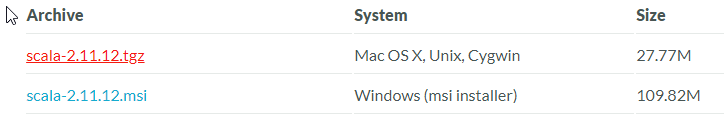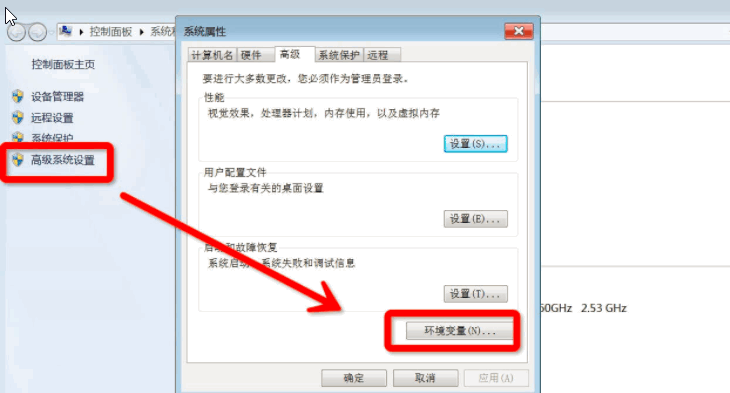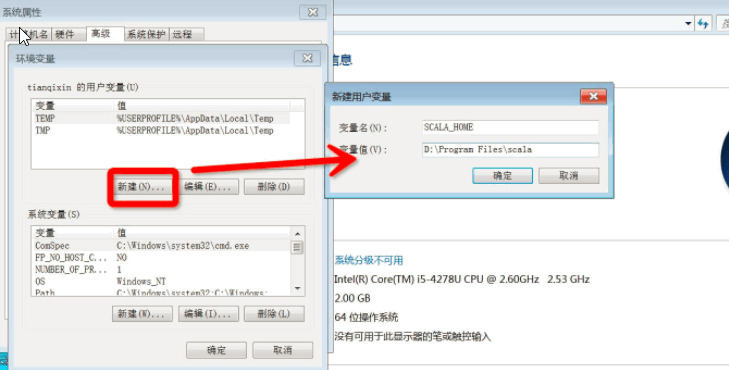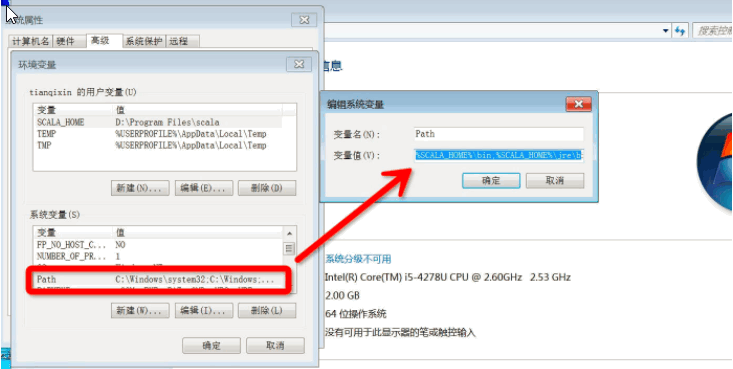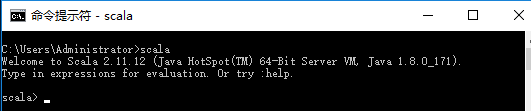### 并发性

Scala使用Actor作为其并发模型，Actor是类似线程的实体，通过邮箱发收消息。Actor可以复用线程，因此在程序中可以使用数百万个Actor,而线程只能创建数千个。在2.10之后的版本中，使用Akka作为其默认Actor实现。

## 三 基础语法

Scala 与 Java 的最大区别是：Scala 语句末尾的分号 ; 是可选的。

• 对象 - 对象有属性和行为。例如：一只狗的状属性有：颜色，名字，行为有：叫、跑、吃等。对象是一个类的实例。

• 类 - 类是对象的抽象，而对象是类的具体实例。

• 方法 - 方法描述的基本的行为，一个类可以包含多个方法。

• 字段 - 每个对象都有它唯一的实例变量集合，即字段。对象的属性通过给字段赋值来创建。

# Scala 包

## 定义包

Scala 使用 package 关键字定义包，在Scala将代码定义到某个包中有两种方式：

### 3.1.1交互式编程

``````\$ scala
Welcome to Scala version 2.11.7 (Java HotSpot(TM) 64-Bit Server VM, Java 1.8.0_31).
Type in expressions to have them evaluated.

scala> 1 + 1
res0: Int = 2

scala> println("Hello World!")
Hello World!

scala>
``````

### 第一种方法

``````package com.runoobclass HelloWorld{    }
``````

### 3.1.2脚本形式

``````object HelloWorld {
/* 这是我的第一个 Scala 程序
* 以下程序将输出'Hello World!'
*/
def main(args: Array[String]) {
println("Hello, world!")
}
}
``````

``````\$ scalac HelloWorld.scala
\$ ls
HelloWorld\$.class    HelloWorld.scala
HelloWorld.class
``````

``````\$ scala HelloWorld
Hello, world!
``````

### 第二种方法

``````package com.runoob {  class HelloWorld{    }}
``````

## 3.2基本语法

Scala 基本语法需要注意以下几点：

• 区分大小写 -  Scala是大小写敏感的，这意味着标识Hello 和 hello在Scala中会有不同的含义。

• 类名 - 对于所有的类名的第一个字母要大写。
如果需要使用几个单词来构成一个类的名称，每个单词的第一个字母要大写。

示例：class MyFirstScalaClass

• 方法名称 - 所有的方法名称的第一个字母用小写。
如果若干单词被用于构成方法的名称，则每个单词的第一个字母应大写。

示例：def myMethodName()

• 程序文件名 - 程序文件的名称应该与对象名称完全匹配(新版本不需要了，但建议保留这种习惯)。
保存文件时，应该保存它使用的对象名称（记住Scala是区分大小写），并追加".scala"为文件扩展名。 （如果文件名和对象名称不匹配，程序将无法编译）。

示例: 假设"HelloWorld"是对象的名称。那么该文件应保存为'HelloWorld.scala"

• def main(args: Array[String]) - Scala程序从main()方法开始处理，这是每一个Scala程序的强制程序入口部分。

## 引用包

Scala 使用 import 关键字引用包。

``````import java.awt.Color  // 引入Color import java.awt._  // 引入包内所有成员 def handler(evt: event.ActionEvent) { // java.awt.event.ActionEvent  ...  // 因为引入了java.awt，所以可以省去前面的部分}
``````

import语句可以出现在任何地方，而不是只能在文件顶部。import的效果从开始延伸到语句块的结束。这可以大幅减少名称冲突的可能性。

``````import java.awt.{Color, Font} // 重命名成员import java.util.{HashMap => JavaHashMap} // 隐藏成员import java.util.{HashMap => _, _} // 引入了util包的所有成员，但是HashMap被隐藏了
``````

`注意`：默认情况下，Scala 总会引入 java.lang._ 、 scala._ 和 Predef._，这里也就解释了，为什么以scala开头的包，在使用时都是省去scala.的。

### 3.2.1标识符

Scala 可以使用两种形式的标志符，字符数字和符号。

Scala 的命名规则采用和 Java 类似的 camel 命名规则，首字符小写，比如 toString。类名的首字符还是使用大写。此外也应该避免使用以下划线结尾的标志符以避免冲突。符号标志符包含一个或多个符号，如+，:，? 等，比如:

``````+ ++ ::: < ?> :->
``````

Scala 内部实现时会使用转义的标志符，比如:-> 使用 \$colon\$minus\$greater 来表示这个符号。因此如果你需要在 Java 代码中访问:->方法，你需要使用 Scala 的内部名称 \$colon\$minus\$greater。

# 数据类型

Scala的数据类型和Java差不多,以下是和Java不同之处.

java... 和java一样,8种基本类型...
Unit 表示无值，和其他语言中void等同。用作不返回任何结果的方法的结果类型。Unit只有一个实例值，写成()。
Null null 或空引用
Nothing Nothing类型在Scala的类层级的最低端；它是任何其他类型的子类型。
Any Any是所有其他类的超类
AnyRef AnyRef类是Scala里所有引用类(reference class)的基类

### 3.2.2Scala 关键字

 abstract case catch class def do else extends false final finally for forSome if implicit import lazy match new null object override package private protected return sealed super this throw trait try true type val var while with yield - : = => <- <: <% >: # @

## 类型的层级关系

### 3.2.3Scala 注释

Scala 类似 Java 支持单行和多行注释。多行注释可以嵌套，但必须正确嵌套，一个注释开始符号对应一个结束符号。注释在 Scala 编译中会被忽略，实例如下：

``````object HelloWorld {
/* 这是一个 Scala 程序
* 这是一行注释
* 这里演示了多行注释
*/
def main(args: Array[String]) {
// 输出 Hello World
// 这是一个单行注释
println("Hello, world!")
}
}
``````

## 多行字符串的表示方法

``````val foo = """菜鸟教程www.runoob.comwww.w3cschool.ccwww.runnoob.com以上三个地址都能访问"""
``````

### 3.2.5换行符

Scala是面向行的语言，语句可以用分号（;）结束或换行符。Scala 程序里,语句末尾的分号通常是可选的。如果你愿意可以输入一个,但若一行里仅 有一个语句也可不写。另一方面,如果一行里写多个语句那么分号是需要的。例如

``````val s = "菜鸟教程"; println(s)
``````

# Scala 变量

### 3.2.6Scala 包

Scala 使用 package 关键字定义包，在Scala将代码定义到某个包中有两种方式：

``````package com.runoob
class HelloWorld
``````

``````package com.runoob {
class HelloWorld
}
``````

Scala 使用 import 关键字引用包。

``````import java.awt.Color  // 引入Color

import java.awt._  // 引入包内所有成员

def handler(evt: event.ActionEvent) { // java.awt.event.ActionEvent
...  // 因为引入了java.awt，所以可以省去前面的部分
}
``````

import语句可以出现在任何地方，而不是只能在文件顶部。import的效果从开始延伸到语句块的结束。这可以大幅减少名称冲突的可能性。

``````import java.awt.{Color, Font}

// 重命名成员
import java.util.{HashMap => JavaHashMap}

// 隐藏成员
import java.util.{HashMap => _, _} // 引入了util包的所有成员，但是HashMap被隐藏了
``````

## 变量声明

``````var myVar : String = "Foo"val myVal : String = "Foo"
``````

++所以，如果在没有指明数据类型的情况下声明变量或常量必须要给出其初始值，否则将会报错。++

## 四 数据类型

Scala 与 Java有着相同的数据类型，下表列出了 Scala 支持的数据类型：

Byte 8位有符号补码整数。数值区间为 -128 到 127
Short 16位有符号补码整数。数值区间为 -32768 到 32767
Int 32位有符号补码整数。数值区间为 -2147483648 到 2147483647
Long 64位有符号补码整数。数值区间为 -9223372036854775808 到 9223372036854775807
Float 32位IEEE754单精度浮点数
Double 64位IEEE754单精度浮点数
Char 16位无符号Unicode字符, 区间值为 U+0000 到 U+FFFF
String 字符序列
Boolean true或false
Unit 表示无值，和其他语言中void等同。用作不返回任何结果的方法的结果类型。Unit只有一个实例值，写成()。
Null null 或空引用
Nothing Nothing类型在Scala的类层级的最低端；它是任何其他类型的子类型。
Any Any是所有其他类的超类
AnyRef AnyRef类是Scala里所有引用类(reference class)的基类

### Scala 支持多个变量同时的声明赋值：

``````val xmax, ymax = 100  // xmax, ymax都声明为100
``````

## 4.1Scala 基础字面量

Scala 非常简单且直观。接下来我们会详细介绍 Scala 字面量。

# 访问修饰符

### 4.1.1整型字面量

``````0
035
21
0xFFFFFFFF
0777L
``````

## 私有

Scala 中的 private 限定符，比 Java 更严格，在嵌套类情况下，外层类甚至不能访问被嵌套类的私有成员。

``````class Outer{    class Inner{        private def f(){            println("f")        }        class InnerMost{            f() // 正确        }    }    (new Inner).f() //错误}
``````

### 4.1.2浮点型字面量

``````0.0
1e30f
3.14159f
1.0e100
.1
``````

## 保护(Protected)

``````package p{    class Super{        protected def f() {            println("f")        }    }    class Sub extends Super{        f()    }    class Other{        (new Super).f() //错误    }}
``````

## 公共

### 4.1.4符号字面量

``````package scala
final case class Symbol private (name: String) {
override def toString: String = "'" + name
}
``````

## 作用域保护

Scala中，访问修饰符可以通过使用限定词强调。格式为:

``````private[x] 或 protected[x]
``````

``````package bobsrocckets{    package navigation{        private[bobsrockets] class Navigator{            protected[navigation] def useStarChart(){}            class LegOfJourney{                private[Navigator] val distance = 100            }            private[this] var speed = 200        }    }    package launch{        import navigation._        object Vehicle{            private[launch] val guide = new Navigator        }    }}
``````

### 4.1.5字符字面量

``````'a'
'u0041'
'n'
't'
``````

# Scala 运算符

### 4.1.6字符串字面量

``````"Hello,nWorld!"
"菜鸟教程官网：www.runoob.com"
``````

## 4.2多行字符串的表示方法

``````val foo = """菜鸟教程
www.runoob.com
www.w3cschool.cc
www.runnoob.com

``````

## 4.3Null 值

Scala.Null和scala.Nothing是用统一的方式处理Scala面向对象类型系统的某些"边界情况"的特殊类型。

Null类是null引用对象的类型，它是每个引用类（继承自AnyRef的类）的子类。Null不兼容值类型。

## 4.4Scala 转义字符

b u0008 退格(BS) ，将当前位置移到前一列
t u0009 水平制表(HT) （跳到下一个TAB位置）
n u000a 换行(LF) ，将当前位置移到下一行开头
f u000c 换页(FF)，将当前位置移到下页开头
r u000d 回车(CR) ，将当前位置移到本行开头
" u0022 代表一个双引号(")字符
' u0027 代表一个单引号（'）字符
\ u005c 代表一个反斜线字符 ''

0 到 255 间的 Unicode 字符可以用一个八进制转义序列来表示，即反斜线‟‟后跟 最多三个八进制。

``````object Test {
def main(args: Array[String]) {
println("HellotWorldnn" );
}
}
``````

``````\$ scalac Test.scala
\$ scala Test
Hello    World
``````

~ 按位取反

## 5.1变量声明

• 一、变量： 在程序运行过程中其值可能发生改变的量叫做变量。如：时间，年龄。
• 二、常量 在程序运行过程中其值不会发生变化的量叫做常量。如：数值 3，字符'A'。

``````var myVar : String = "Foo"
var myVar : String = "Too"
``````

``````val myVal : String = "Foo"
``````

# 流程语句

## 5.2变量类型声明

``````var VariableName : DataType [=  Initial Value]

val VariableName : DataType [=  Initial Value]
``````

## 5.3变量类型引用

``````var myVar = 10;
val myVal = "Hello, Scala!";
``````

## 5.4Scala 多个变量声明

Scala 支持多个变量的声明：

``````val xmax, ymax = 100  // xmax, ymax都声明为100
``````

``````scala> val pa = (40,"Foo")
pa: (Int, String) = (40,Foo)
``````

# Scala 函数

Scala 有函数和方法，二者在语义上的区别很小。

Scala 方法是类的一部分，而函数是一个对象可以赋值给一个变量。换句话来说在类中定义的函数即是方法。

## 六 访问修饰符

Scala 访问修饰符基本和Java的一样，分别有：private，protected，public。

Scala 中的 private 限定符，比 Java 更严格，在嵌套类情况下，外层类甚至不能访问被嵌套类的私有成员。

## 函数声明

``````def functionName  : [return type]
``````

## 6.1私有成员

``````class Outer{
class Inner{
private def f(){println("f")}
class InnerMost{
f() // 正确
}
}
(new Inner).f() //错误
}
``````

(new Inner).f( ) 访问不合法是因为 f 在 Inner 中被声明为 private，而访问不在类 Inner 之内。

Java中允许这两种访问，因为它允许外部类访问内部类的私有成员。

## 函数定义

``````def functionName  : [return type] = {   function body   return [expr]}
``````

``````object add{   def addInt( a:Int, b:Int ) : Int = {      var sum:Int = 0      sum = a + b      return sum   }}
``````

Scala的灵活性,使的函数定义的方法多种多样:
1、规规矩矩的写法，带有等号、大括号和返回值类型的形式

``````def myFunc : Int = {    //something}def myFunc : Unit = {    //something}
``````

2、非unit返回值的情形下，省略返回值，让程序根据代码块，自行判断。`注意`，这里等号还是要的

``````def myFunc = {    //something}
``````

3、unit返回值的情况下，直接省略返回值类型和等号

``````def myFunc(var p1 : Int) {    //something    // return unit}
``````

4、函数只有一行的情形下省略返回值和大括号

``````def max2(x: Int, y: Int) = if  x else y def greet() = println("Hello, world!")
``````

## 6.2保护成员

``````package p{
class Super{
protected def f() {println("f")}
}
class Sub extends Super{
f()
}
class Other{
(new Super).f() //错误
}
}
``````

## 函数调用

Scala 提供了多种不同的函数调用方式：

``````functionName
``````

``````[instance.]functionName
``````

## 6.3公共成员

Scala中，如果没有指定任何的修饰符，则默认为 public。这样的成员在任何地方都可以被访问。

``````class Outer {
class Inner {
def f() { println("f") }
class InnerMost {
f() // 正确
}
}
(new Inner).f() // 正确因为 f() 是 public
}
``````

### 函数传名调用(call-by-name)

Scala的解释器在解析函数参数(function arguments)时有两种方式：

• 传值调用（call-by-value）：先计算参数表达式的值，再应用到函数内部；
• 传名调用（call-by-name）：将未计算的参数表达式直接应用到函数内部;

``````object Test {   def main(args: Array[String]) {        delayed;   }   def time() = {      println("获取时间，单位为纳秒")      System.nanoTime   }   def delayed( t: => Long ) = {      println("在 delayed 方法内")      println("参数： " + t)      t   }}
``````

``````在 delayed 方法内获取时间，单位为纳秒参数： 241550840475831获取时间，单位为纳秒
``````

``````获取时间，单位为纳秒在 delayed 方法内参数： 241550840475831
``````

## 6.4作用域保护

Scala中，访问修饰符可以通过使用限定词强调。格式为:

``````private[x]

protected[x]
``````

``````package bobsrocckets{
private[bobsrockets] class Navigator{
class LegOfJourney{
private[Navigator] val distance = 100
}
private[this] var speed = 200
}
}
package launch{
object Vehicle{
private[launch] val guide = new Navigator
}
}
}
``````

### 指定函数参数名

``````object Test {   def main(args: Array[String]) {        printInt;   }   def printInt( a:Int, b:Int ) = {      println("Value of a : " + a );      println("Value of b : " + b );   }}
``````

## 七 运算符

Scala 含有丰富的内置运算符，包括以下几种类型：

• 算术运算符

• 关系运算符

• 逻辑运算符

• 位运算符

• 赋值运算符

### 可变参数

Scala 允许你指明函数的最后一个参数可以是重复的，即我们不需要指定函数参数的个数，可以向函数传入可变长度参数列表。
Scala 通过在参数的类型之后放一个星号来设置可变参数。例如：

``````object Test {   def main(args: Array[String]) {        printStrings("Runoob", "Scala", "Python");   }   def printStrings( args:String* ) = {      var i : Int = 0;      for( arg <- args ){         println("Arg value[" + i + "] = " + arg );         i = i + 1;      }   }}
``````

## 7.1算术运算符

+ 加号 A + B 运算结果为 30
- 减号 A - B 运算结果为 -10
* 乘号 A * B 运算结果为 200
/ 除号 B / A 运算结果为 2
% 取余 B % A 运算结果为 0

``````object Test {
def main(args: Array[String]) {
var a = 10;
var b = 20;
var c = 25;
var d = 25;
println("a + b = " + (a + b) );
println("a - b = " + (a - b) );
println("a * b = " + (a * b) );
println("b / a = " + (b / a) );
println("b % a = " + (b % a) );
println("c % a = " + (c % a) );

}
}
``````

``````\$ scalac Test.scala
\$ scala Test
a + b = 30
a - b = -10
a * b = 200
b / a = 2
b % a = 0
c % a = 5
``````

## 7.2关系运算符

== 等于 (A == B) 运算结果为 false
!= 不等于 (A != B) 运算结果为 true
> 大于 (A > B) 运算结果为 false
< 小于 (A < B) 运算结果为 true
>= 大于等于 (A >= B) 运算结果为 false
<= 小于等于 (A <= B) 运算结果为 true

``````object Test {
def main(args: Array[String]) {
var a = 10;
var b = 20;
println("a == b = " + (a == b) );
println("a != b = " + (a != b) );
println("a > b = " + (a > b) );
println("a < b = " + (a < b) );
println("b >= a = " + (b >= a) );
println("b <= a = " + (b <= a) );
}
}
``````

``````\$ scalac Test.scala
\$ scala Test
a == b = false
a != b = true
a > b = false
a < b = true
b >= a = true
b <= a = false
``````

### 默认参数值

``````def addInt( a:Int=5, b:Int=7 ) : Int = { }
``````

## 7.3逻辑运算符

&& 逻辑与 (A && B) 运算结果为 false
|| 逻辑或 (A || B) 运算结果为 true
! 逻辑非 !(A && B) 运算结果为 true

``````object Test {
def main(args: Array[String]) {
var a = true;
var b = false;

println("a && b = " + (a&&b) );

println("a || b = " + (a||b) );

println("!(a && b) = " + !(a && b) );
}
}
``````

``````\$ scalac Test.scala
\$ scala Test
a && b = false
a || b = true
!(a && b) = true
``````

### 高阶函数（Higher-Order Function）

Scala 中允许使用高阶函数,高阶函数可以使用其他函数作为参数，或者使用函数作为输出结果。

## 7.4位运算符

p q p & q p | q p ^ q
0 0 0 0 0
0 1 0 1 1
1 1 1 1 0
1 0 0 1 1

``````A = 0011 1100

B = 0000 1101

-------位运算----------

A&B = 0000 1100

A|B = 0011 1101

A^B = 0011 0001

~A  = 1100 0011
``````

Scala 中的按位运算法则如下：

& 按位与运算符 (a & b) 输出结果 12 ，二进制解释： 0000 1100
| 按位或运算符 (a | b) 输出结果 61 ，二进制解释： 0011 1101
^ 按位异或运算符 (a ^ b) 输出结果 49 ，二进制解释： 0011 0001
~ 按位取反运算符 (~a ) 输出结果 -61 ，二进制解释： 1100 0011， 在一个有符号二进制数的补码形式。
<< 左移动运算符 a << 2 输出结果 240 ，二进制解释： 1111 0000
>> 右移动运算符 a >> 2 输出结果 15 ，二进制解释： 0000 1111
>>> 无符号右移 A >>>2 输出结果 15, 二进制解释: 0000 1111

``````object Test {
def main(args: Array[String]) {
var a = 60;           /* 60 = 0011 1100 */
var b = 13;           /* 13 = 0000 1101 */
var c = 0;

c = a & b;            /* 12 = 0000 1100 */
println("a & b = " + c );

c = a | b;            /* 61 = 0011 1101 */
println("a | b = " + c );

c = a ^ b;            /* 49 = 0011 0001 */
println("a ^ b = " + c );

c = ~a;               /* -61 = 1100 0011 */
println("~a = " + c );

c = a << 2;           /* 240 = 1111 0000 */
println("a << 2 = " + c );

c = a >> 2;           /* 15 = 1111 */
println("a >> 2  = " + c );

c = a >>> 2;          /* 15 = 0000 1111 */
println("a >>> 2 = " + c );
}
}
``````

``````\$ scalac Test.scala
\$ scala Test
a & b = 12
a | b = 61
a ^ b = 49
~a = -61
a << 2 = 240
a >> 2  = 15
a >>> 2 = 15
``````

## 7.5赋值运算符

= 简单的赋值运算，指定右边操作数赋值给左边的操作数。 C = A + B 将 A + B 的运算结果赋值给 C
+= 相加后再赋值，将左右两边的操作数相加后再赋值给左边的操作数。 C += A 相当于 C = C + A
-= 相减后再赋值，将左右两边的操作数相减后再赋值给左边的操作数。 C -= A 相当于 C = C - A
*= 相乘后再赋值，将左右两边的操作数相乘后再赋值给左边的操作数。 C *= A 相当于 C = C * A
/= 相除后再赋值，将左右两边的操作数相除后再赋值给左边的操作数。 C /= A 相当于 C = C / A
%= 求余后再赋值，将左右两边的操作数求余后再赋值给左边的操作数。 C %= A is equivalent to C = C % A
<<= 按位左移后再赋值 C <<= 2 相当于 C = C << 2
>>= 按位右移后再赋值 C >>= 2 相当于 C = C >> 2
&= 按位与运算后赋值 C &= 2 相当于 C = C & 2
^= 按位异或运算符后再赋值 C ^= 2 相当于 C = C ^ 2
|= 按位或运算后再赋值 C |= 2 相当于 C = C | 2

``````object Test {
def main(args: Array[String]) {
var a = 10;
var b = 20;
var c = 0;

c = a + b;
println("c = a + b  = " + c );

c += a ;
println("c += a  = " + c );

c -= a ;
println("c -= a = " + c );

c *= a ;
println("c *= a = " + c );

a = 10;
c = 15;
c /= a ;
println("c /= a  = " + c );

a = 10;
c = 15;
c %= a ;
println("c %= a  = " + c );

c <<= 2 ;
println("c <<= 2  = " + c );

c >>= 2 ;
println("c >>= 2  = " + c );

c >>= 2 ;
println("c >>= a  = " + c );

c &= a ;
println("c &= 2  = " + c );

c ^= a ;
println("c ^= a  = " + c );

c |= a ;
println("c |= a  = " + c );
}
}
``````

``````\$ scalac Test.scala
\$ scala Test
c = a + b  = 30
c += a  = 40
c -= a = 30
c *= a = 300
c /= a  = 1
c %= a  = 5
c <<= 2  = 20
c >>= 2  = 5
c >>= a  = 1
c &= 2  = 0
c ^= a  = 10
c |= a  = 10
``````

1 () [] 左到右
2 ! ~ 右到左
3 * / % 左到右
4 + - 左到右
5 >> >>> << 左到右
6 > >= < <= 左到右
7 == != 左到右
8 & 左到右
9 ^ 左到右
10 | 左到右
11 && 左到右
12 || 左到右
13 = += -= *= /= %= >>= <<= &= ^= |= 右到左
14 , 左到右

### 匿名函数

Scala 中定义匿名函数的语法很简单，箭头左边是参数列表，右边是函数体。

``````var mul = (x: Int, y: Int) => x*y
``````

## 八 IF...ELSE 语句

Scala IF...ELSE 语句是通过一条或多条语句的执行结果（True或者False）来决定执行的代码块。### 偏应用函数

Scala 偏应用函数是一种表达式，你不需要提供函数需要的所有参数，只需要提供部分，或不提供所需参数。

``````import java.util.Dateobject Test {   def main(args: Array[String]) {      val date = new Date      log(date, "message1" )      Thread.sleep      log(date, "message2" )      Thread.sleep      log(date, "message3" )   }   def log(date: Date, message: String)  = {     println(date + "----" + message)   }}
``````

``````Mon Dec 02 12:52:41 CST 2013----message1Mon Dec 02 12:52:41 CST 2013----message2Mon Dec 02 12:52:41 CST 2013----message3
``````

``````import java.util.Dateobject Test {   def main(args: Array[String]) {      val date = new Date      val logWithDateBound = log(date, _ : String)      logWithDateBound("message1" )      Thread.sleep      logWithDateBound("message2" )      Thread.sleep      logWithDateBound("message3" )   }   def log(date: Date, message: String)  = {     println(date + "----" + message)   }}
``````

## 8.1if 语句

if 语句有布尔表达式及之后的语句块组成。

if 语句的语法格式如下：

``````if(布尔表达式)
{
// 如果布尔表达式为 true 则执行该语句块
}
``````

``````object Test {
def main(args: Array[String]) {
var x = 10;

if( x < 20 ){
println("x < 20");
}
}
}
``````

``````\$ scalac Test.scala
\$ scala Test
x < 20
``````

### 函数柯里化(Function Currying)

``````def add(x:Int,y:Int)=x+y
``````

``````def add = x + y
``````

## 8.2if...else 语句

if 语句后可以紧跟 else 语句，else 内的语句块可以在布尔表达式为 false 的时候执行。

if...else 的语法格式如下：

``````if(布尔表达式){
// 如果布尔表达式为 true 则执行该语句块
}else{
// 如果布尔表达式为 false 则执行该语句块
}
``````

``````object Test {
def main(args: Array[String]) {
var x = 30;

if( x < 20 ){
println("x 小于 20");
}else{
println("x 大于 20");
}
}
}
``````

``````\$ scalac Test.scala
\$ scala Test
x 大于 20
``````

## Scala 闭包

``````object Test {     def main(args: Array[String]) {        println( "muliplier value = " +  multiplier        println( "muliplier value = " +  multiplier     }     var factor = 3     val multiplier =  => i * factor  }
``````

## 8.3if...else if...else 语句

if 语句后可以紧跟 else if...else 语句，在多个条件判断语句的情况下很有用。

if...else if...else 语法格式如下：

``````if(布尔表达式 1){
// 如果布尔表达式 1 为 true 则执行该语句块
}else if(布尔表达式 2){
// 如果布尔表达式 2 为 true 则执行该语句块
}else if(布尔表达式 3){
// 如果布尔表达式 3 为 true 则执行该语句块
}else {
// 如果以上条件都为 false 执行该语句块
}
``````

``````object Test {
def main(args: Array[String]) {
var x = 30;

if( x == 10 ){
println("X 的值为 10");
}else if( x == 20 ){
println("X 的值为 20");
}else if( x == 30 ){
println("X 的值为 30");
}else{
println("无法判断 X 的值");
}
}
}
``````

``````\$ scalac Test.scala
\$ scala Test
X 的值为 30
``````

# Scala 字符串

``````object Test {   val greeting: String = "Hello,World!"   def main(args: Array[String]) {      println( greeting )   }}
``````